# Tied in a Mathematical Knot

Over the next several weeks, sites across the globe will celebrate mathematics through puzzles, magic and fun. For some, "mathematical fun" is an oxymoron. However, these events are for more than the mathematically gifted as they celebrate the life and accomplishments of Martin Gardner. It has been said that Gardner turned thousands of children into mathematicians and thousands of mathematicians into children. In case there isn't one of these Celebration of Mind events near you, let's have one, of sorts, on The Huffington Post through this article.

To begin, get some string, preferably slippery, like a lanyard and tangle it up -- either haphazardly or methodically, your choice. Then, tape the ends together. My tangle is seen below.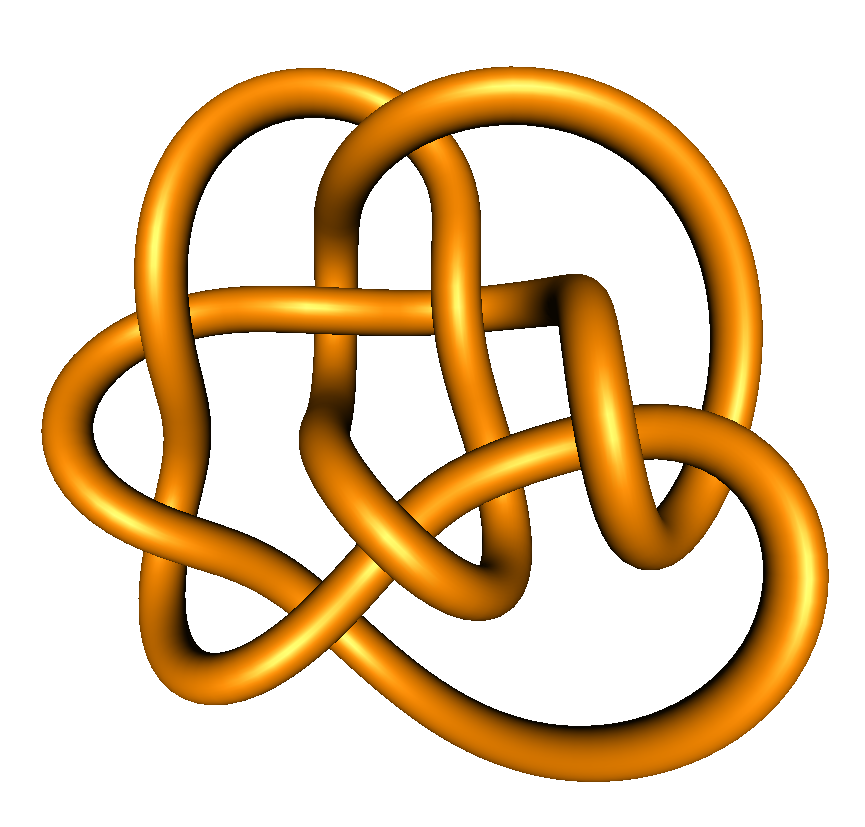Untangle it as much as you can. What do you get? Can you untangle it so it forms a loop? Why or why not? Could I have formed a loop without breaking the string from my tangle pictured above?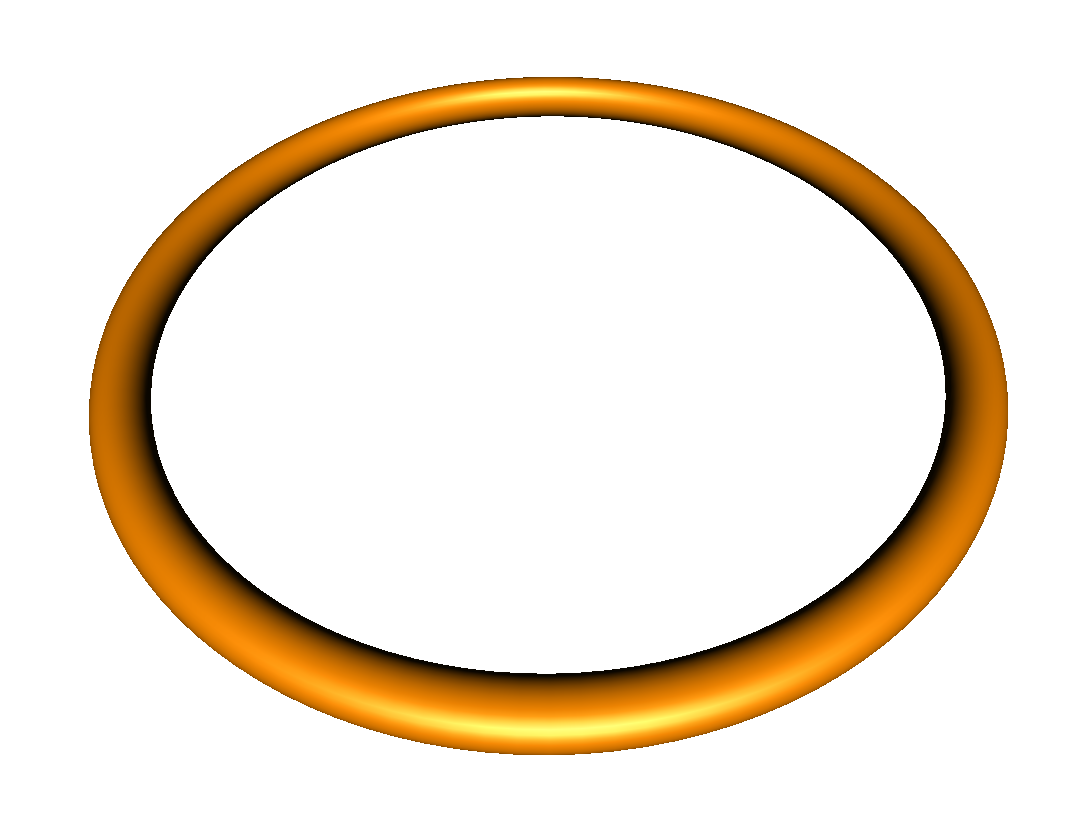Math often looks at equality. For example, x2 + x = 0 implies x = -1 or x = 0. Another important idea is equivalence. We have such a notion in our daily thinking. For example, a kitchen stool and recliner can both be thought of as chairs even though they are not exactly the same. The field of topology, sometimes called rubber sheet geometry, looks at the equivalence of objects.

Let's touch on an aspect of this field of math by playing a game with a piece of rope. This time, the rope can shrink or stretch as needed. The rules are simple. We'll have some initial and target shapes. Can we move from the initial to target shape without detaching or attaching the ends of the rope? If it is possible to do so, the shapes are equivalent, else they are not.

Suppose, we put our string in the shape of the letter L as seen below? If we take each of the other pictured letters as our target shape, which are equivalent to the letter L?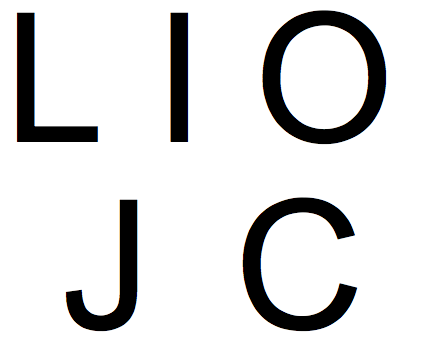If you think of the string as a caterpillar, it need only straighten itself to move from the L to the I. It need only to curve its lower-half and curve its whole body to form the J and C. The O presents an issue as there is simply no way for a rope with 2 exposed endpoints to form a shape with no endpoints. So, L is equivalent to all the letters except the O in the picture above.

Let's try again. Suppose you form a single cord with 3 ends into the letter T as seen below. Which of the other letters are mathematically equivalent?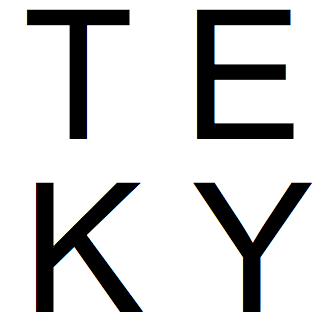It doesn't take much to get from the T to the Y. It can take some contortions of the mind to see how to get from the T to the E, although some find it easier to imagine going from the Y to the E. Seeing that you cannot get from the T, E or Y to the K can be difficult as you, of course, must imagine what is impossible. One helpful insight is to notice that the K has 4 endpoints. The other letters have 3. You cannot get from 3 to 4 endpoints without detaching the string or cord at some point. So, the letter K is not equivalent to the other letters.

Let's end by considering the mathematical equivalence of a donut to an egg. Suppose some day in the future, an astronaut is traveling through space and crashes on a distant planet. She has only a compass (which we will assume points north at all times) and the very large amount of debris from the fallen ship. By leaving a sufficiently long trail of debris (assuming nothing on the planet moves the trail), how could the astronaut determine whether the planet forms an egg or a donut-shaped planet as seen below? Note, the planet is cover in clouds so she can't, if she is in the interior of the donut, look up and see the planet above her! An egg shape does not contain a hole and the donut does. This difference indicates, like the number of endpoints in the letters T and K, that the two shapes are not equivalent. See if you can devise a way to note their difference by drawing a line along the planet, in this case, with the debris of the ship.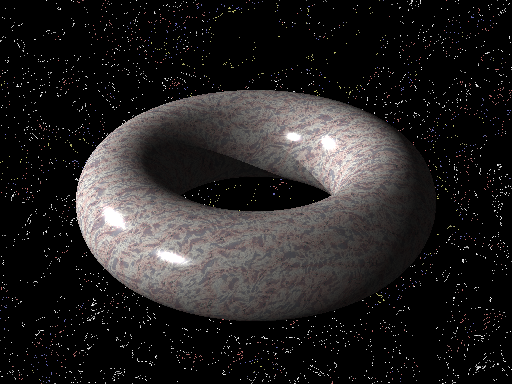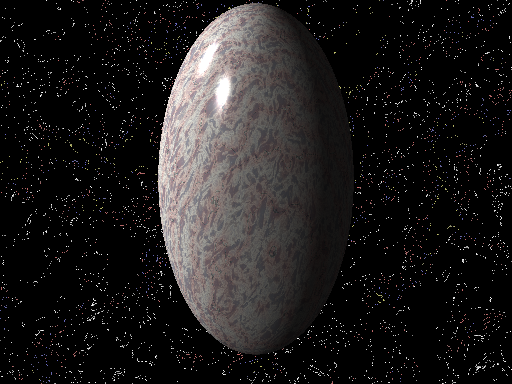As you twist your thinking to contemplate these concepts, how good are you at this type of math? Some may find this easier than algebra, for instance. Others may find it harder. If "mathematical fun" seems like an oxymoron, it may simply be that you haven't been exposed to a branch of mathematical study that fits you. There are many types of math. Where should you look? The publications of Martin Gardner a good starting spot; you'll find math in the form of puzzles, magic, and much more.

Acknowledgement: Thanks to Henry Segerman for creating the images of the tangled knot and the loop. Thanks also to Anna Weltman for her insights on this article.